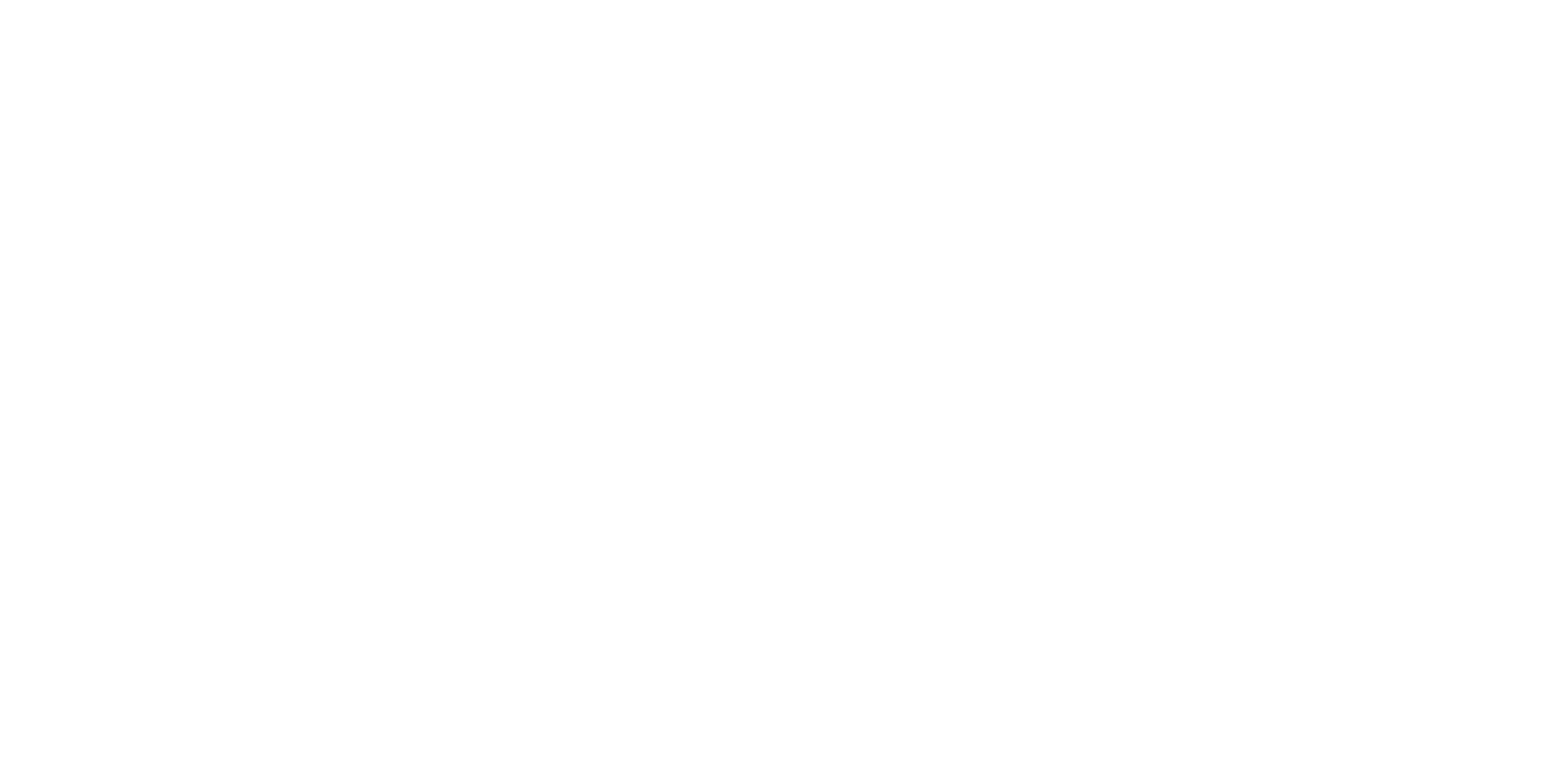Kuulchat

Online Education Resource Portal, its all free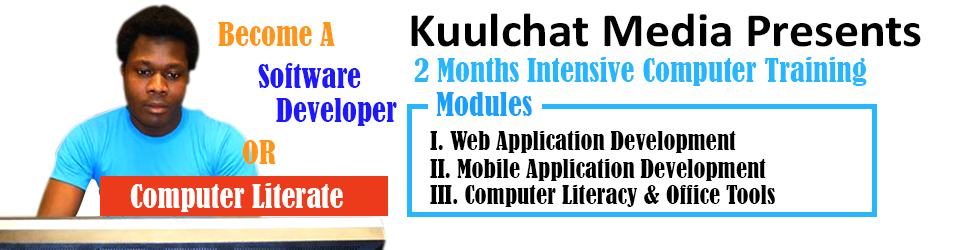BECE MATHEMATICS 2019

#### OBJECTIVE TEST

1.

Given that A = {2,4,6,8,10} and B = {4,8,12}, find AB.

A.

{4,8}

B.

{2,8,12}

C.

{4,6,8,12}

D.

{2,4,6,8,10,12}

2.

Express 0.000344 in standard form.

A.

3.44 x 10-6

B.

3.44 x 10-5

C.

3.44 x 10-4

D.

3.44 x 10-3

3.

Which of the following numbers is the largest?

A.

-70

B.

-50

C.

-3

D.

-2

4.

Correct 0.024561 to three significant figures.

A.

0.03

B.

0.025

C.

0.0245

D.

0.0246

5.

Simplify: (75x73)÷76

A.

79

B.

74

C.

73

D.

72

6.

How many lines of symmetry has a square?

A.

0

B.

1

C.

2

D.

4

7.

Solve the equation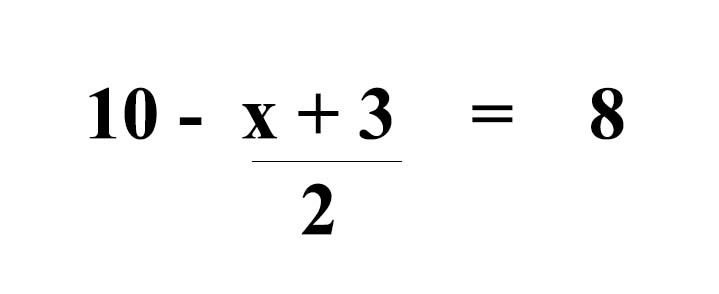A.

-9

B.

-3

C.

1

D.

15

8.

Factorize: kx+2xt-4k-8t.

A.

(k-2t)(x+4)

B.

(k+2t)(x+4)

C.

(k+t)(x-4)

D.

(k+2t)(x-4)

9.

There are 12 boys and 18 girls in a class

Find the fraction of boys in the class.

A.

B.

C.

D.

¾

10.

Express 30% as a fraction in its lowest term.

A.

7⁄10

B.

3⁄20

C.

7⁄20

D.

3⁄10

11.

Make k the subject of the relation, ky - k = y2

A.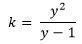B.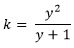C.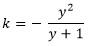D.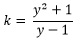12.

The mean of the numbers 5, 2x,4 and 3 is 5. Find the value of x.

A.

3

B.

4

C.

5

D.

8

13.

Find the rule of the mapping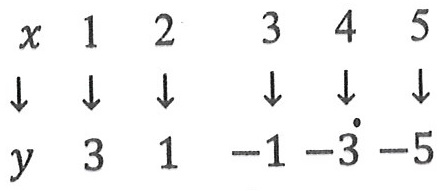A.

y = 2x + 2

B.

y = -2x + 2

C.

y = 4x

D.

y = -2x + 5

14.

The two sides of a parallelogram are 4.8 m and 7.2 m long. Find its perimeter.

A.

48.0 m

B.

34.6 m

C.

24.0 m

D.

17.3 m

15.

A tank in the form of a cuboid has length 6 m and breadth 4 m. If the volume of the tank is 36 m3, find the height.

A.

0.67 m

B.

1.5 m

C.

1.8 m

D.

5.0 m

16.

If the bearing of A from B is 240o, find the bearing of B from A

A.

040o

B.

060o

C.

120o

D.

300o

17.

Find the truth set of the inequality 2y + 5 < 4y - 5.

A.

{y:y > 5}

B.

{y:y < 5}

C.

{y:y > 1}

D.

{y:y > 0}

18.

Find the gradient of the straight line which passes through the points (-3,4) and (3,-2).

A.

2

B.

1

C.

-2

D.

-1

19.

If 6:8 = r:48, find the value of r

A.

36

B.

34

C.

14

D.

12

20.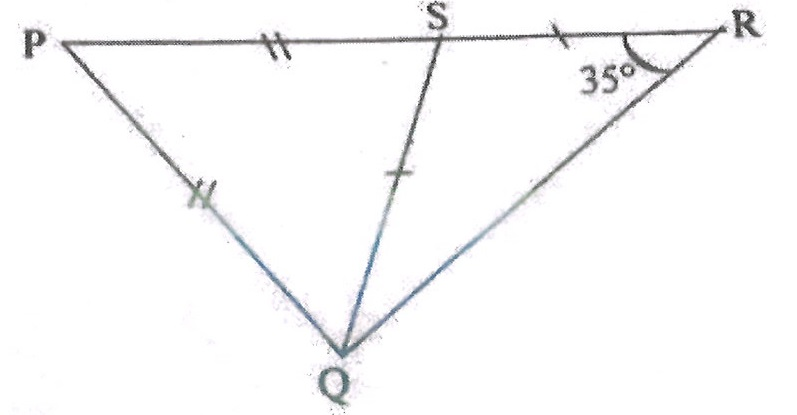Find ∠QPS in the diagram.

A.

70o

B.

40o

C.

35o

D.

20o

21.

A man travelled a distance of 8 km in an hour. How long will it take him to cover a distance of 12 km, travelling at the same speed?

A.

1⅓ hrs

B.

1½ hrs

C.

1¾ hrs

D.

2 hrs

22.

A number is selected at random from: 25,26,27,28,...,35. Find the probability that the number selected is a prime number.

A.

6⁄11

B.

3⁄11

C.

2⁄11

D.

1⁄11

23.

Express 12/25 in decimal fraction.

A.

0.0408

B.

0.048

C.

0.408

D.

0.48

24.

Find the diameter of a circle whose circumference is 88 cm. [Take π = 22⁄7]

A.

14 cm

B.

22 cm

C.

28 cm

D.

82 cm

25.

When twelve is subtracted from three times a certain number and the result is divided by four, the answer is eighteen. Find the number.

A.

84

B.

40

C.

28

D.

20

26.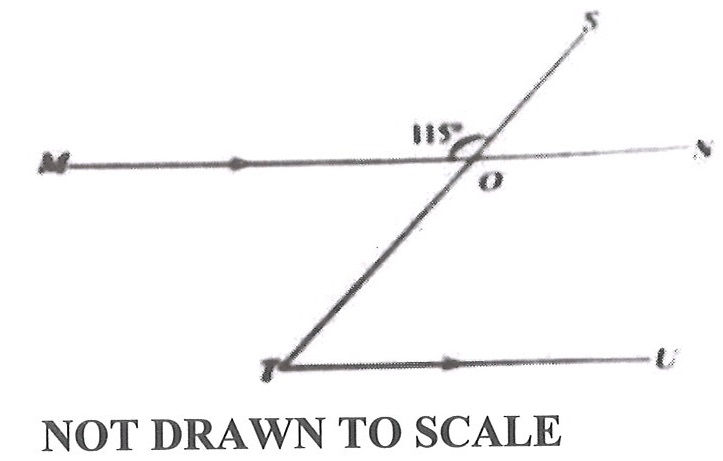In the diagram, line MN is parallel to line TU, line TS cuts line MN at O and ∠MOS = 115o. Find ∠OTU.

A.

65o

B.

55o

C.

45o

D.

25o

27.

Given that r =and t =,

find r + t

A.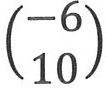B.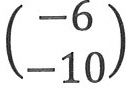C.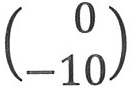D.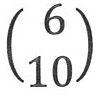28.

A trader sold 90 oranges at 3 for GH₵ 0.75. How much did she get from selling all the oranges?

A.

GH₵ 22.50

B.

GH₵ 67.50

C.

GH₵ 75.00

D.

GH₵ 225.50

29.

Express 72 as a product of prime factors.

A.

23 x 32

B.

22 x 33

C.

22 x 32

D.

2 x 3

30.

Simplify: 3a x 24ab.

A.

27ab2

B.

27a2b

C.

72ab2

D.

72a2b

31.

Simplify: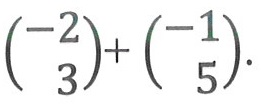A.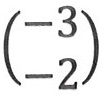B.C.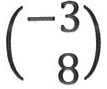D.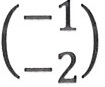32.

Multiply 247 by 32.

A.

6916

B.

7804

C.

7904

D.

1235

33.

Evaluate: (0.07 x 0.02) ÷ 14.

A.

0.01

B.

0.001

C.

0.0001

D.

0.00001

34.

In a class of 23 students, the girls were 7 more than the boys. How many boys were in the class?

A.

8

B.

15

C.

16

D.

30

35.

Express 30 minutes as a percentage of 3 hours 20 minutes

A.

12.5 %

B.

15 %

C.

16⅔ %

D.

20 %

36.

Find the Least Common Multiple (L.C.M) of 2, 3 and 5.

A.

6

B.

12

C.

24

D.

30

37.

The simple interest on GH₵ 450.00 for 4 years is GH₵ 45.00, find the rate of interest

A.

2.5 %

B.

10 %

C.

25 %

D.

6.5 %

38.

Find the median of the following numbers: 46, 68, 34, 37, 76 and 81.

A.

35.5

B.

57

C.

67

D.

68

39.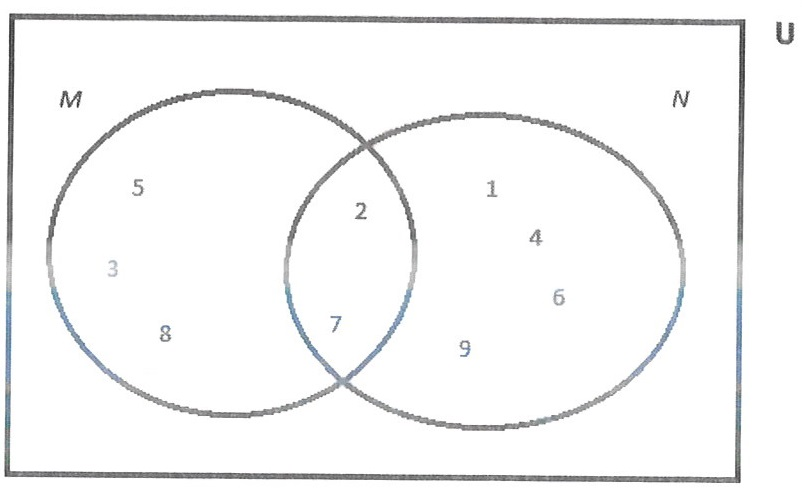In the Venn diagram M and N are the subsets of the universal set U.

Use this information to answer the question below.

Find MN

A.

{7}

B.

{2,7}

C.

{3,5,8}

D.

{1,2,3,4,5,6,7,8,9}

40.In the Venn diagram M and N are the subsets of the universal set U.

Use this information to answer the question below.

How many members are in the set N?

A.

2

B.

3

C.

4

D.

6

#### THEORY QUESTIONS

1.

a

Given that X = {whole numbers from 4 to 13} and Y = {multiples of 3 between 2 and 20}, find XY.

b

Find the Least Common Multiple (L.C.M) of the following numbers: 3,5 and 9.

c

If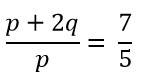, find the value of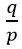,

Show Solution
2.

a

Solve: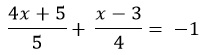.

b

The ratio of boys to girls in a school is 12:25. If there are 120 boys.

i) how many girls are in the school?
ii) what is the total number of boys and girls in the school?

c

Simplify: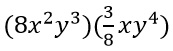Show Solution
3.

a

In an examination 60 candidates passed Integrated Science or Mathematics. If 15 passed both subjects and 9 more passed Mathematics than Integrated Science, find the:
i) number of candidates who passed in each subject;
ii) probability that a candidate passed exactly one subject.

b

Factorize: xy + 6x + 3y + 18

Show Solution
4.

a

Express 250 % as a fraction in its lowest term.

b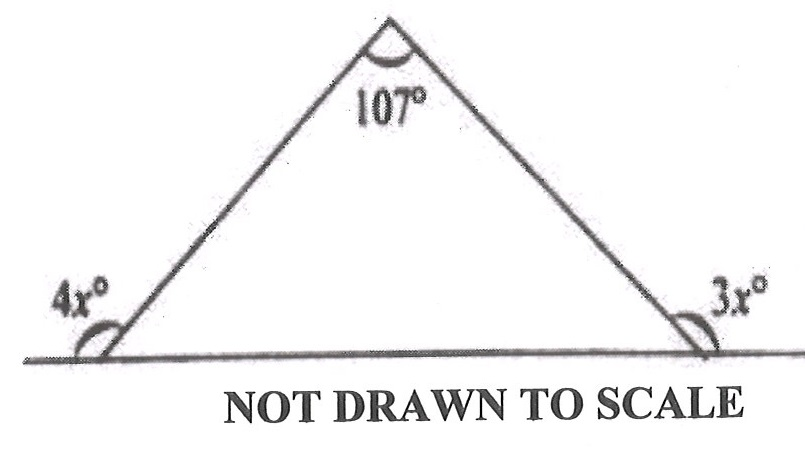Use the diagram to find the value of x.

c

Simplify:d

If q =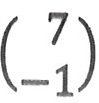and r =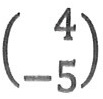find (q + r).

Show Solution